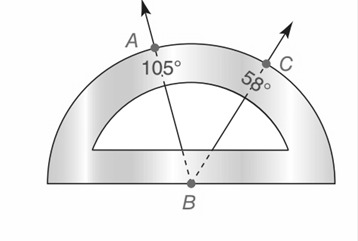Chapter P.CT, Problem 13CTElementary Geometry For College St...

7th Edition
Alexander + 2 others
ISBN: 9781337614085

Solutions

Chapter
SectionElementary Geometry For College St...

7th Edition
Alexander + 2 others
ISBN: 9781337614085
Textbook Problem

Use the protractor with measures as indicted to find ∠ A B CTo determine

To find:

The value of x.

Explanation

Given,

Calculation:

Let us take the initial angle start with D and end with E, then the diagram becomes

DBC=58 and EBA=105

To find the ABC, subtracting the given two angles

Ther

Still sussing out bartleby?

Check out a sample textbook solution.

See a sample solution

The Solution to Your Study Problems

Bartleby provides explanations to thousands of textbook problems written by our experts, many with advanced degrees!

Get Started

Multiply: 30,0104080

Elementary Technical Mathematics

The polar coordinates of the point P in the figure at the right are:

Study Guide for Stewart's Multivariable Calculus, 8th

True or False: converges.

Study Guide for Stewart's Single Variable Calculus: Early Transcendentals, 8th